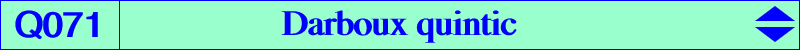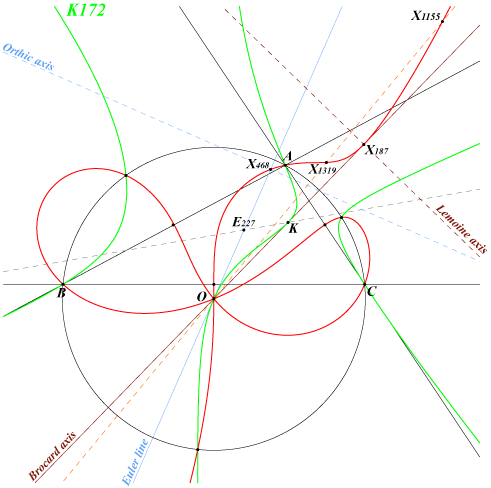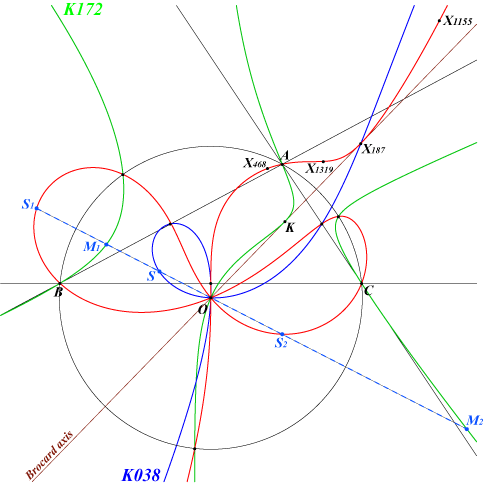too complicated to be written here. Click on the link to download a text file.X(3), X(30), X(187), X(468), X(1155), X(1319) midpoints of ABC points on the Thomson cubic or K172 and on the circumcircle inverses of the points on K172 in the circumcircle (see below)Q071 is the isogonal transform of the Darboux septic Q001 and the inversive image of K172 in the circumcircle. Q071 is a bicircular quintic with a real asymptote passing through X(74) and parallel to the Euler line, that of the Neuberg cubic. It meets the circumcircle at the same points as the Thomson cubic and K172. The tangents at the three points apart A, B, C are concurrent at X(11284), a point on the Euler line and on the lines X(6)X(373), X(394)X(576). Q071 contains the midpoints of ABC and the tangents at these points are the perpendicular bisectors of ABC. Q071 is related to the Darboux cubic in the following way : denote by PaPbPc the pedal triangle of point P. The circles PAPa, PBPb, PCPc form a pencil if and if P lies on the Darboux cubic (together with the line at infinity) (Hyacinthos #6329 and related messages). In this case, they have two common (real) points P, P' and P' lies on Q071, the Darboux quintic. When P lies on the Darboux cubic, its pedal triangle is a cevian triangle with perspector Q on the Lucas cubic. The isogonal conjugate Q* of Q lies on K172 and P' is the inverse of Q* in the circumcircle.Any line L passing through O meets the quintic at O (which is a triple point) and two other points S1, S2 which are the inverses of the points M1, M2 on the cubic K172. The midpoint S of S1S2 lies on the Stammler strophoid K038. When L is the Brocard axis, these points coincide : M1 = M2 = K and S = S1 = S2 = X(187).